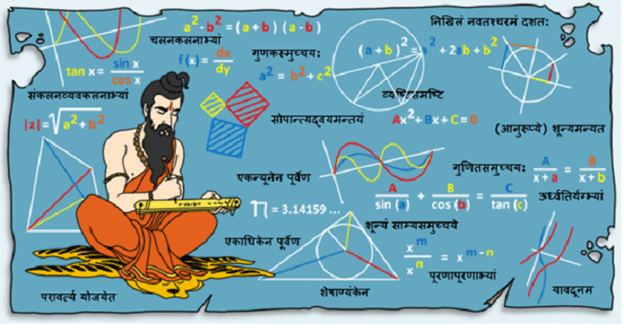# What is difference between ABACUS and Vedic Maths?

People always confuse between ABACUS and Vedic Maths. Few people think both are same, as both teach to do faster calculations. This is a myth that ABACUS and Vedic Maths are nearly same.

What is ABACUS? ABACUS is a complete 360 degree brain development program for kids. It helps kids to develop their focus, concentration and many other such characteristics. It is just a byproduct that kids learn to do fast mathematical calculations.

ABACUS is a wooden frame which has rods and beads. We all know that we forget numbers but not images so easily. In ABACUS, kids picturize and remember ABACUS frame in the mind which helps them focus on the position of beads to calculate any arithmetic equation faster in just fraction of seconds.

Whereas, Vedic Maths is a collection of techniques (Sutras) to solve mathematical arithmetic in easy and faster way. It consists of 16 formulas (sutras) and 13 sub-formulas (sub sutras) which can be used for problems involved in arithmetic, algebra, geometry, calculus, conics.